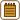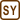## Nitrogen in a Vessel

### Task number: 2173

One mole of nitrogen is enclosed in a vessel. Average translational kinetic energy of the nitrogen molecules is 3.5 kJ. Determine the most probable speed of nitrogen molecules after increasing temperature in the vessel by 200 K.

• #### Hint 1

Using the formula for average molecular kinetic energy of a gas $$\bar{E}_k$$, express the temperature T1 of nitrogen before heating.

• #### Hint 2: The Most Probable Speed

To calculate the most probable speed vp of the heated nitrogen molecules, use the following relation:

$v_p=\sqrt{\frac{2RT_2}{M_m}},$

where Mm is the molar mass of nitrogen, T2 is the temperature of the heated nitrogen and R is the molar gas constant.

• #### Notation

 $$\bar{E}_k=3.5\,\mathrm{kJ}=3500\,\mathrm{J}$$ average translational kinetic energy of nitrogen molecules n = 1 mol amount of nitrogen ΔT = 200 K increase in temperature vp = ? the most probable speed

From the Tables:

 Mm = 28 g mol−1 = 0.028 kg mol−1 molar mass of nitrogen R = 8.31 J mol−1K−1 molar gas constant
• #### Analysis

First we express the temperature of nitrogen before heating from the formula for the average kinetic energy of a gas, according to which the energy is directly proportional to the thermodynamic temperature. After that we add the temperature change to the calculated initial temperature and obtain the temperature of the heated nitrogen, which we subsequently substitute in the formula for the most probable molecular speed.

• #### Solution

The average kinetic energy $$\bar{E}_k$$ of any gas is directly proportional to its thermodynamic temperature T. The following relation applies

$\bar{E}_k=\frac{3}{2}nRT,$

where n is the amount of the gas and R is the molar gas constant.

From this formula we can easily determine temperature T1 of the nitrogen before heating:

$T_1=\frac{2\bar{E}_k}{3nR}.$

For temperature T2 of the heated nitrogen it holds true

$T_2=T_1+\mathrm{\Delta}T=\frac{2\bar{E}_k}{3nR}+\mathrm{\Delta}T.$

Finally, to calculate the most probable speed vp of the nitrogen molecules, we use the known formula

$v_p=\sqrt{\frac{2RT_2}{M_m}},$

where Mm is the molar mass of the nitrogen, and substitute for the obtained expression of temperature T2:

$v_p=\sqrt{\frac{2R}{M_m}\left(\frac{2\bar{E}_k}{3nR}+\mathrm{\Delta} T\right)}.$
• #### Numerical Substitution

$v_p=\sqrt{\frac{2R}{M_m}\left(\frac{2\bar{E}_k}{3nR}+\mathrm{\Delta} T\right)}=\sqrt{\frac{2\cdot{8.31}}{0.028}\cdot\left(\frac{2\cdot{3500}}{3\cdot{1}\cdot{8.31}}+200\right)}\,\mathrm{m\,s}^{-1}$ $v_p\dot{=}530\,\mathrm{m\,s}^{-1}$

Just out of interest let's also try to determine the most probable speed of the nitrogen molecules before heating:

$v_p=\sqrt{\frac{4\bar{E}_k}{3nM_m}}=\sqrt{\frac{4\cdot{3500}}{3\cdot{1}\cdot{0.028}}}\,\mathrm{m\,s}^{-1}$ $v_p\dot{=}410\,\mathrm{m\,s}^{-1}$
• #### Answer

The most probable speed of the nitrogen molecules after its temperature been increased is approximately 530 ms−1.

• #### Comment

Try to compare the determined speed of the nitrogen molecules with the speeds we encounter in everyday life.

By comparing the calculated value with the speed of the air under normal conditions, we obtain the approximate value of the Mach number 1.6. So the speed is supersonic.